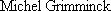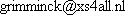This pages shows the basic physics formulae for ballistic projectiles. The projectiles are assumed to be shot at a 45 degrees (for best range) or 90 degrees (for best height) angle, from ground level.

The formulas assume there is no air resistance. Also, for very high velocity projectiles (>1000 m/s) these formulas are not appropiate anymore.

When shot upwards:

 max. height = 0.5 * v * v / g Example: at 36 Km/hour (10 m/s), this gives max height=0.5*10*10/9.8=5.1 meter. time of flight = 2 * v / g Example: at 36 Km/hour (10 m/s), this gives time of flight=2.04 seconds launch velocity = 0.5 * g * (time of flight) Example: 5 seconds time of flight gives 24.5 m/s (88 Km/hour) launch velocity = square root (2 * g * height) Example: 10 meter height gives 14 m/s (50 Km/h) range = 0

When shot at a 45 degree angle:

 max. height = 0.25 * v * v / g Example: at 36 Km/hour (10 m/s), this gives max height=0.25*10*10/9.8=2.5 meter. time of flight = 1.41 * v / g Example: at 36 Km/hour (10 m/s), this gives time of flight=1.44 seconds launch velocity = square root ( g * range ) Examle: if range=35 m, velocity=18.5 m/s (67 Km/h) launch velocity = 0.71 * g * (time of flight) Example: 5 seconds time of flight gives 34.6 m/s (124 Km/hour) launch velocity = 2 * square root ( g * max height) Example: 10 meter height gives 19.8 m/s (71 Km/h) range = v * v / g or 4 * max. height Example: 100 Km/h (27.8 m/s) gives a range of 79 meter range = 0.5 * (time of flight)2 Example: 5.5 sec flight time gives a range of 148 meter. Warning: mind the air resistance

v=velocity
g=gravity (between 9.78 and 9.83 m/s2, or 32.2 feet/sec2)

1 m/s=3.6 Km/hour
1 m/s=3.281 feet/second=2.24 miles/hour
Energy = force * distance = mass * g * height = 0.5 * mass * v * v

Note that all of the above formulas are irrespective of the units you use; put feet and pounds in and you get feet and pounds out.

(c),, 16 Apr 2001